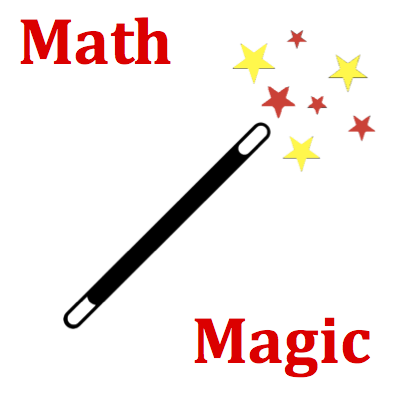# Circular and Satellite Motion - Mission CG6 Detailed HelpSuppose that an object weighs 90 Newtons on the surface of the earth (a distance of R from its center). If the same object is located a distance of 2R above the Earth's surface (a distance of 3R from its center), then the force of gravity upon it would be ____ Newtons. (Note: Your numerical values are randomly selected and may vary from those shown above.)Newton's Law of Universal Gravitation: All objects with mass attract each other with a gravitational force. The magnitude of the force is directly related to the product of the masses and inversely related to the square of the separation distance between their centers.The gravitational force is inversely proportional to the square of the separation distance. The inverse nature of the law means that if the distance is increased, then the gravitational force will be decreased. The inverse squarenature of the law means the gravitational force will be decreased by the square of the factor by which the separation distance is increased. So if the separation distance becomes two times bigger, then the gravitational force will become four times (22) smaller. The new gravitational force would be one-fourth the original value. If the separation distance becomes three times bigger, then the gravitational force will become nine times (32) smaller. The new gravitational force would be one-ninth the original value.